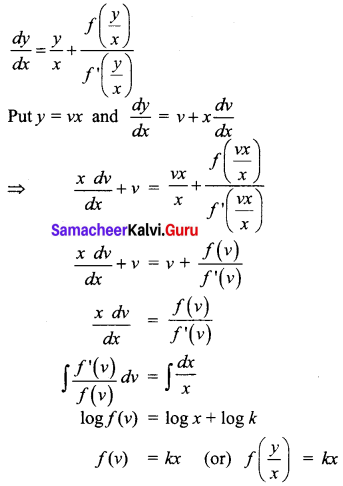# Samacheer Kalvi 12th Business Maths Solutions Chapter 4 Differential Equations Ex 4.6

Students can download 12th Business Maths Chapter 4 Differential Equations Ex 4.6 Questions and Answers, Samacheer Kalvi 12th Business Maths Book Solutions Guide Pdf helps you to revise the complete Tamilnadu State Board New Syllabus and score more marks in your examinations.

## Tamilnadu Samacheer Kalvi 12th Business Maths Solutions Chapter 4 Differential Equations Ex 4.6

Question 1.
The degree of the differential equation $$\frac{d^{4} y}{d x^{4}}-\left(\frac{d^{2} y}{d x^{2}}\right)^{4}+\frac{d y}{d x}=3$$ is _________
(a) 1
(b) 2
(c) 3
(d) 4
(a) 1
Hint:
Since the power of $$\frac{d^{4} y}{d x^{4}}$$ is 1

Question 2.
The order and degree of the differential equation $$\sqrt{\frac{d^{2} y}{d x^{2}}}=\sqrt{\frac{d y}{d x}+5}$$ are respectively
(a) 2 and 3
(b) 3 and 2
(c) 2 and 1
(d) 2 and 2
(c) 2 and 1
Hint:
Squaring both sides, we get $$\frac{d^{2} y}{d x^{2}}=\frac{d y}{d x}+5$$
So order = 2, degree = 1

Question 3.
The order and degree of the differential equation $$\left(\frac{d^{2} y}{d x^{2}}\right)^{\frac{3}{2}}-\sqrt{\left(\frac{d y}{d x}\right)}-4=0$$ are respectively.
(a) 2 and 6
(b) 3 and 6
(c) 1 and 4
(d) 2 and 4
(a) 2 and 6
Hint: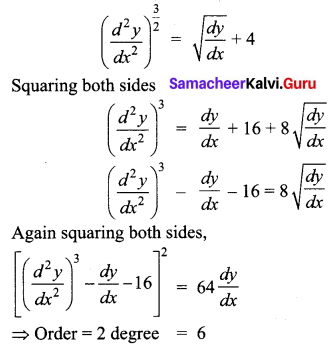Question 4.
The differential equation $$\left(\frac{d x}{d y}\right)^{3}+2 y^{\frac{1}{2}}=x$$ is _________
(a) of order 2 and degree 1
(b) of order 1 and degree 3
(c) of order 1 and degree 6
(d) of order 1 and degree 2
(b) of order 1 and degree 3

Question 5.
The differential equation formed by eliminating a and b from y = aex + be-x is _______
(a) $$\frac{d^{2} y}{d x^{2}}-y=0$$
(b) $$\frac{d^{2} y}{d x^{2}}-\frac{d y}{d x}=0$$
(c) $$\frac{d^{2} y}{d x^{2}}=0$$
(d) $$\frac{d^{2} y}{d x^{2}}-x=0$$
(a) $$\frac{d^{2} y}{d x^{2}}-y=0$$
Hint: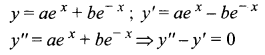Question 6.
If y = cx + c – c3 then its differential equation is ______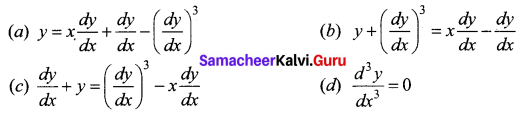(a) $$y=x \frac{d y}{d x}+\frac{d y}{d x}-\left(\frac{d y}{d x}\right)^{3}$$

Question 7.
The integrating factor of the differential equation $$\frac{d x}{d y}$$ + Px = Q is _____
(a) e∫Pdx
(b) ∫Pdx
(c) ∫Pdy
(d) e∫Pdy
(d) e∫Pdy

Question 8.
The complementary function of (D2 + 4) y = e2x is _______
(a) (Ax + B) e2x
(b) (Ax + B) e-2x
(c) A cos 2x + B sin 2x
(d) Ax-2x + Be2x
(c) A cos 2x + B sin 2x
Hint:
A.E = m2 + 4 = 0 ⇒ m = ±2i
C.F = e0x (A cos 2x + B sin 2x)

Question 9.
The differential equation of y = mx + c is (m and c are arbitrary constants).
(a) $$\frac{d^{2} y}{d x^{2}}=0$$
(b) y = x $$\frac{d y}{d x}$$
(c) x dy + y dx = 0
(d) y dx – x dy = 0
(a) $$\frac{d^{2} y}{d x^{2}}=0$$

Question 10.
The particular integral of the differential equation $$\frac{d^{2} y}{d x^{2}}-8 \frac{d y}{d x}+16 y=2 e^{4 x}$$ is ________
(a) $$\frac{x^{2} e^{4 x}}{2 !}$$
(b) $$\frac{e^{4 x}}{2 !}$$
(c) x2 e4x
(d) xe4x
(c) x2 e4x
Hint: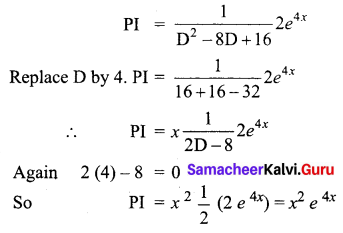Question 11.
Solution of $$\frac{d x}{d y}$$ + px = 0
(a) x = cepy
(b) x = ce-py
(c) x = py + c
(d) x = cy
(b) x = ce-py
Hint: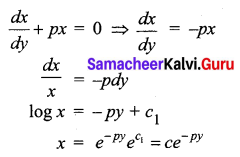Question 12.
If sec2 x is an integrating factor of the differential equation $$\frac{d y}{d x}$$ + Py = Q then P = _____
(a) 2 tan x
(b) sec x
(c) cos2 x
(d) tan2 x
(a) 2 tan x
Hint: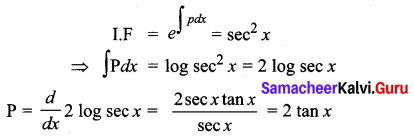Question 13.
The integrating factor of x$$\frac{d y}{d x}$$ – y = x2 is _____
(a) $$\frac{-1}{x}$$
(b) $$\frac{1}{x}$$
(c) log x
(d) x
(b) $$\frac{1}{x}$$
Hint: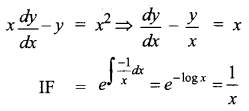Question 14.
The solution of the differential equation $$\frac{d y}{d x}$$ + Py = Q where P and Q are the function of x is ______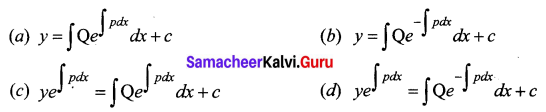(c) $$y e^{\int p d x}=\int \mathrm{Q} e^{\int p d x} d x+c$$

Question 15.
The differential equation formed by eliminating A and B from y = e-2x (A cos x + B sin x) is _______
(a) y2 – 4y1 + 5y = 0
(b) y2 + 4y1 – 5y = 0
(c) y2 – 4y1 – 5y = 0
(d) y2 + 4y1 + 5y = 0
(d) y2 + 4y1 + 5y = 0
Hint: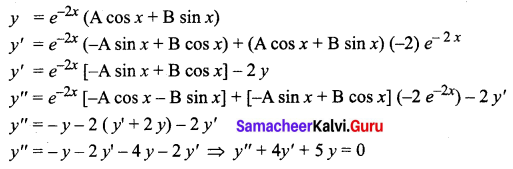Question 16.
The particular integral of the differential equation f(D) y = eax where f(D) = (D – a)2 ________
(a) $$\frac{x^{2}}{2} e^{2 x}$$
(b) xeax
(c) $$\frac{x}{2} e^{2 x}$$
(d) x2 e2x
(a) $$\frac{x^{2}}{2} e^{2 x}$$

Question 17.
The differential equation of x2 + y2 = a2 is _____
(a) x dy + y dx = 0
(b) y dx – x dy = 0
(c) x dx – y dx = 0
(d) x dx + y dy = 0
(d) x dx + y dy = 0
Hint:
x2 + y2 = a2
⇒ 2x + 2y $$\frac{d y}{d x}$$ = 0
⇒ x dx + y dy = 0

Question 18.
The complementary function of $$\frac{d^{2} y}{d x^{2}}-\frac{d y}{d x}=0$$ is ______
(a) A + B ex
(b) (A + B) ex
(c) (Ax + B) ex
(d) (Aex + B)
(a) A + B ex
Hint:
A.E is m2 – m = 0
⇒ m(m – 1) = 0
⇒ m = 0, 1
CF is Ae0x + Bex = A + Bex

Question 19.
The P.I of (3D2 + D – 14)y = 13e2x is _______
(a) $$\frac{x}{2}$$ e2x
(b) x e2x
(c) $$\frac{x^{2}}{2}$$ e2x
(d) 13xe2x
(b) xe2x
Hint: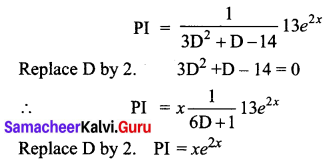Question 20.
The general solution of the differential equation $$\frac{d y}{d x}$$ = cos x is _______
(a) y = sin x + 1
(b) y = sin x – 2
(c) y = cos x + c, c is an arbitrary constant
(d) y = sin x + c, c is an arbitrary constant
(d) y = sin x + c, c is an arbitrary constant

Question 21.
A homogeneous differential equation of the form $$\frac{d y}{d x}=f\left(\frac{y}{x}\right)$$ can be solved by making substitution
(a) y = vx
(b) v = yx
(c) x = vy
(d) x = v
(a) y = vx

Question 22.
A homogeneous differential equation of the form $$\frac{d x}{d y}=f\left(\frac{x}{y}\right)$$ can be solved by making substitution,
(a) x = vy
(b) y = vx
(c) y = v
(d) x = v
(a) x = vy

Question 23.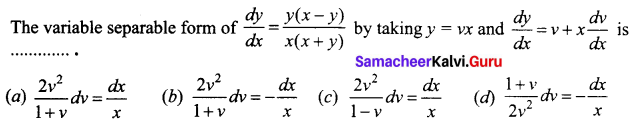(d) $$\frac{1+v}{2 v^{2}} d v=-\frac{d x}{x}$$
Hint: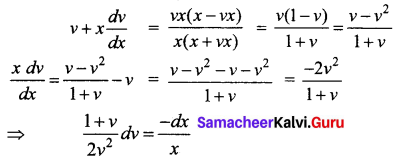Question 24.
Which of the following is the homogeneous differential equation?
(a) (3x – 5) dx = (4y – 1) dy
(b) xy dx – (x3 + y3) dy = 0
(c) y2 dx + (x2 – xy – y2) dy = 0
(d) (x2 + y) dx = (y2 + x) dy
The solution of the differential equation $$\frac{d y}{d x}=\frac{y}{x}+\frac{f\left(\frac{y}{x}\right)}{f^{\prime}\left(\frac{y}{x}\right)}$$ is ______
(a) f($$\frac{y}{x}$$) = kx
(b) x f($$\frac{y}{x}$$) = k
(c) f($$\frac{y}{x}$$) = ky
(d) y f($$\frac{y}{x}$$) = k
(a) f($$\frac{y}{x}$$) = kx